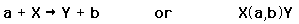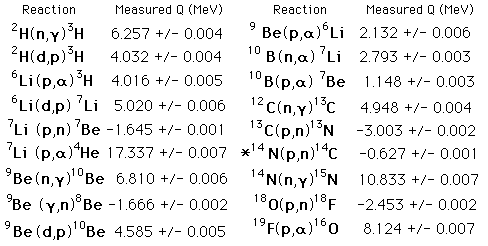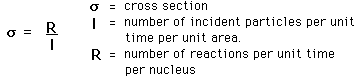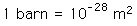# Nuclear Reactions

Many kinds of nuclear reactions occur in response to the absorption of particles such as neutrons or protons. Other types of reactions may involve the absorption of gamma rays or the scattering of gamma rays. Of particular note is the resonant absorption of gamma rays in the Mossbauer effect. Specific nuclear reactions can be written down in a manner similar to chemical reaction equations. If a target nucleus X is bombarded by a particle a and results in a nucleus Y with emitted particle b, this is commonly written in one of two ways.We can characterize the energetics of the reaction with a reaction energy Q, defined as the energy released in the reaction. The Q is positive if the total mass of the products is less than that of the projectile and target, indicating that the total nuclear binding energy has increased. The probability of a given type of nuclear reaction taking place is often stated as a "cross section".

 Table of examples
Index
HyperPhysics***** Nuclear Go Back

# Some Nuclear Reactions* The nuclear reaction in the atmosphere which produces carbon-14 for radiocarbon dating.

Data from C. W. Li, W. Whaling, W. A. Fowler, and C. C. Lauritson, Physical Review 83:512 (1951)

Index
HyperPhysics***** Nuclear Go Back

# Nuclear Cross Section

To characterize the probability that a certain nuclear reaction will take place, it is customary to define an effective size of the nucleus for that reaction, called a cross section. The cross section is defined byThe cross section has the units of area and is on the order of the square of the nuclear radius. A commonly used unit is the barn:A standard old story was that in the early days of the field, a particular cross section turned out to be much bigger than expected. An experimenter exclaimed "Why, that's as big as a barn!" and a unit name was born.

 Scattering Cross Section
Index
HyperPhysics***** Nuclear Go Back